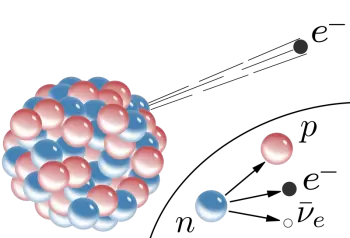# Beta Decay: What Are Beta Particles and Beta Radiation TypesA beta particle (β) is a high-energy electron or positron shot out due to a radioactive event. Beta rays or beta radiation is a form of ionizing radiation emitted by certain types of radioactive nuclei.

Beta decay occurs when, in a nucleus high atomic number (which means too many neutrons or protons), one of the neutrons or protons is converted into the other.

The kinetic energy of beta particles can be from zero to maximum energy, which can be several tens of MeV. The speed of the particles in beta rays is close to the speed of light.

They have lower kinetic energy than alpha particles because although they have a high speed, they have very little mass.

Its rest mass equals the proton's mass divided by 1830 (almost 2000 times smaller). Beta particles have a negative charge and are deflected by electric and magnetic fields.

Initially, beta radiation was not recognized for what it was: an electron beam. The beta particle (β) was identified as an electron when, applying the theory of relativity, the mass of a moving electron was calculated to coincide with that of the b particle. It has a mass more significant than that of an electron at rest.

By Fajans law, if an atom emits a beta particle, its electrical charge increases, and the atomic mass number does not change. That means the number of nucleons (protons plus neutrons) remains constant.

The interaction of beta particles with matter generally has a ten times greater range of action and an ionizing power equal to one-tenth compared to the interaction of alpha particles. On the other hand, beta radiation is more ionizing than gamma-ray radiation.

A layer of a few millimeters of aluminum can stop beta particles. However, such a thin shield is not enough for absolute protection.

## How Do Beta Rays Affect the Human Body?

Beta particles emitted by a radioactive element are moderately penetrant into living tissue and can cause skin burns and spontaneous DNA mutations. However, like alpha particles, beta particles are more dangerous when ingested or inhaled than on skin contact.

Beta radiation sources can be used in radiation therapy to kill cancer cells.

## What Is Beta Decay?

Beta decay is a type of radioactive decay in which an unstable atom emits so-called beta (β) particles, which are high-energy particles ejected from an unstable atomic nucleus.

There are two forms of beta decay:

• In negative beta decay (β- decay), an electron is emitted. In a beta β- decay process, a neutron is converted into a proton, an electron, and an electron antineutrino (the neutrino's antiparticle). Thus, when the beta particle is an electron, the decay is called beta-minus decay.

• Positive beta decay (β+ decay): when positive beta decay occurs, it generates a positron emission observable in proton-rich nuclei. A proton interacts with an electron antineutrino to obtain a neutron and a positron (direct decay of the proton into the positron has not yet been observed).

Beta-plus decay can only occur inside nuclei when the absolute value of the binding energy of the parent nucleus is lower than that of the daughter. The difference in the energy is due to the reaction of converting a proton into a neutron, a positron, and a neutrino and the kinetic energy of these particles.

In beta minus decay, a neutron decays into a proton, an electron, and an antineutrino: n Æ p + e - +. In beta plus decay, a proton decays into a neutron, a positron, and a neutrino: p Æ n + e+ +n.

Enrico Fermi's beta decay theory establishes the presence of the neutrino that prevents the atom and the beta particle from going in opposite directions according to the energy conservation law.

Beta decays also include electron capture. In this type of decay, the atom's nucleus captures an electron from its electron shell and emits an electron neutrino.

## Uses of Beta Particles

Beta ray emission is used in different fields of science:

• For example, in medicine, beta particles are used in some medical treatments, such as bone or eye cancer.

• Radioactive isotopes of iodine are used in treating thyroid cancer because iodine is taken up by the thyroid and emits beta particles that kill cancer cells.

• In nuclear medicine, they are also used as markers.

• Quality control of the thickness of some materials, such as paper.

• Obtaining phosphorescent light for emergencies in which electricity is not available.

Author:

Published: March 8, 2019
Last review: July 14, 2022